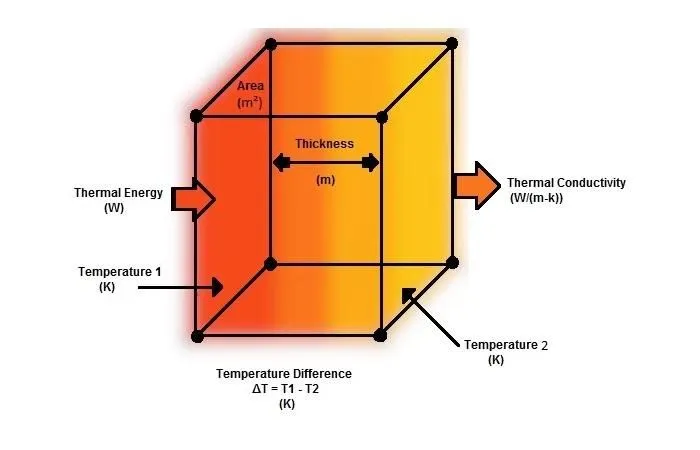## Shopping Cart

Order total 0€Terminology of some basic coefficient which are related to the thermal insulation of a building.

Terminology of some basic coefficient which are related to the thermal insulation of a building.

## THERMO-PHYSICAL PROPERTIES OF BUILDING MATERIALS

Coefficient of Thermal Conductivity (L): It is the amount of heat (in watts) that passes through the opposite sides of a material, one metre thick, when the temperature difference between these surfaces is equal to one Kelvin degree 1⁰K.

The coefficient (L) of a material is measured in watts per metre and degrees Kelvin (W/mk) and is influenced by the nature of the material itself, its structure, temperature, humidity and pressure.

Thermal conductivity is high in materials called thermally conductive, such as metals. Also, it is low in materials called thermally insulating, so the lower the specific coefficient of a material, the better thermal insulation exists.

Coefficient of Thermal Transmittance (U): It is the amount of heat (in watts) that passes through a square meter of a building element of a certain thickness d in a certain time interval of one hour, when there is a temperature difference of one degree Kelvin between the two surfaces. It measures the easement with which heat passes through a material or system within the ambits which are mentioned before.

The U-value is measured in watts per square meter and Kelvin degree (W/m²K) and is mathematically expressed by the formula U=1/R where R is the coefficient of thermal resistance which we will see below.

The lower the specific coefficient of a building element, material or layers are, of materials, the better the thermal insulation we have.

The specific coefficient is affected by the thickness combined to the coefficient (λ) of the materials in a system.

Coefficient of Thermal Resistance (R): It is the inverse of the coefficient of Thermal Transmittance. It measures the difficulty (resistance of the measured elements) of passing heat through a material or layers of materials (system) with a temperature difference on both sides equal to one degree Kelvin.

The coefficient R is measured in square meters times by Kelvin degrees per watt (m² K/W) and is expressed mathematically by the formula R=d/λ where d is the thickness of the material and λ is the coefficient of thermal conductivity.

The higher (inversely to the previous coefficients) the coefficient R of a material is, the better its thermal insulation we have.

Thermal capacity (C): It is the amount of heat stored by a structural element of a space that is heated (or air-conditioned) when the temperature difference between its surfaces is always equal to 1⁰C.

The stored amount of heat, is increased by the big temperature difference between the structural element and air surrounding it, and is as big as the specific heat capacity and mass of the element.

In case where heat capacity is increased, the rate of heating and cooling of the space is slow and on hot days there is reduced heating of the space. The increased thermal capacity helps to balance the temperature during sudden heat-cooling cycles.

Coefficient of thermal radiation emittance (e): It is the ratio of the thermal radiation emitted by a body to the thermal radiation of a black body (values from 0 to 1).

Useful advice: The best measure of the thermal insulation performance of a material is the coefficient of thermal resistance R. To compare materials or systems do the simple operation of dividing material thickness (d)/coefficient L.

### Are you ready for the next step?

#### Insulation - Thermal insulation - Waterproofing

Cookies are necessary for the proper functioning of monodomiki.gr and for enriching your online experience.